# 一、catalan数由来和性质

## 1）由来

catalan数（卡塔兰数）取自组合数学中一个常在各种计数问题中出现的数列。以比利时的数学家欧仁·查理·卡塔兰 (18141894)命名。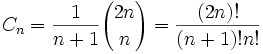令其为h(n)的话，满足h(n)= h(0)*h(n-1)+h(1)*h(n-2) + ... + h(n-1)h(0) (n>=2)

我们从中取出的Cn就叫做第nCatalan数，前几个Catalan数是：1, 1, 2, 5, 14, 42, 132, 429, 1430, 4862, 16796, 58786, 208012, 742900, 2674440, 9694845, 35357670, …咋看之下没什么特别的，但是Catalan数却是许多计数问题的最终形式。

## 2）性质

1Catalan数的基本公式就是上面列出的式子，但是却有一些变形和具体的性质：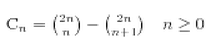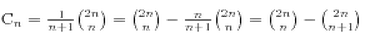2、有如下的递推式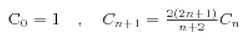3、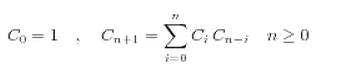4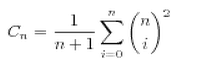# 二、Catalan数的程序求解

1. //函数功能: 计算Catalan数列的第n项
2. //函数参数: 项数n
3. //返回值:   第n个Catalan数
4. long Catalan(int n)
5. {
6. if(n <= 1)
7. return 1;
8. long *h = new long[n+1]; //保存临时结果
9. h = h = 1;        //h(0)和h(1)
10. for(int i = 2; i <= n; i++)    //依次计算h(2),h(3)...h(n)
11. {
12. h[i] = 0;
13. for(int j = 0; j < i; j++) //根据递归式计算 h(i)= h(0)*h(i-1)+h(1)*h(i-2) + ... + h(i-1)h(0)
14. h[i] += (h[j] * h[i-1-j]);
15. }
16. long result = h[n]; //保存结果
17. delete [] h;       //注意释放空间
18. return result;
19. }

# 三、Catalan数的应用场景和笔试面试中出现过的题目

## 1、括号匹配问题

n对括号有多少种匹配方式？

n对括号相当于有2n个符号，n个左括号、n个右括号，可以设问题的解为f(2n)。第0个符号肯定为左括号，与之匹配的右括号必须为第2i+1字符。因为如果是第2i个字符，那么第0个字符与第2i个字符间包含奇数个字符，而奇数个字符是无法构成匹配的。

通过简单分析，f(2n)可以转化如下的递推式 f(2n) = f(0)*f(2n-2) + f(2)*f(2n - 4) + ... + f(2n - 4)*f(2) + f(2n-2)*f(0)。简单解释一下，f(0) * f(2n-2)表示第0个字符与第1个字符匹配，同时剩余字符分成两个部分，一部分为0个字符，另一部分为2n-2个字符，然后对这两部分求解。f(2)*f(2n-4)表示第0个字符与第3个字符匹配，同时剩余字符分成两个部分，一部分为2个字符，另一部分为2n-4个字符。依次类推。

假设f(0) = 1，计算一下开始几项，f(2) = 1, f(4) = 2, f(6) = 5。结合递归式，不难发现f(2n) 等于h(n)

## 2、进栈出栈问题

这个与加括号的很相似，进栈操作相当于是左括号，而出栈操作相当于右括号。n个数的进栈次序和出栈次序构成了一个含2n个数字的序列。第0个数字肯定是进栈的数，这个数相应的出栈的数一定是第2i+1个数。因为如果是2i，那么中间包含了奇数个数，这奇数个肯定无法构成进栈出栈序列。

设问题的解为f(2n)， 那么f(2n) = f(0)*f(2n-2) + f(2)*f(2n-4) + f(2n-2)*f(0)f(0) * f(2n-2)表示第0个数字进栈后立即出栈，此时这个数字的进栈与出栈间包含的数字个数为0，剩余为2n-2个数。f(2)*f(2n-4)表示第0个数字进栈与出栈间包含了2个数字，相当于1 2 2 1，剩余为2n-4个数字。依次类推。

假设f(0) = 1，计算一下开始几项，f(2) = 1, f(4) = 2, f(6) = 5。结合递归式，不难发现f(2n) 等于h(n)

## 3、二叉树的种类问题

n个节点构成的二叉树，共有多少种情形？

可以这样考虑，根肯定会占用一个结点，那么剩余的n-1个结点可以有如下的分配方式，T(0, n-1),T(1, n-2),...T(n-1, 0)，设T(i, j)表示根的左子树含i个结点，右子树含j个结点。

设问题的解为f(n)，那么f(n) = f(0)*f(n-1) + f(1)*f(n-2) + .......+ f(n-2)*f(1) + f(n-1)*f(0)。假设f(0) = 1，那么f(1) = 1, f(2) = 2, f(3) = 5。结合递推式，不难发现f(n)等于h(n)

## 4、网格路径问题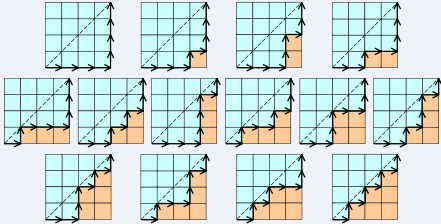## 5、凸多边形分割问题

以凸多边形的一边为基，设这条边的2个顶点为AB。从剩余顶点中选1个，可以将凸多边形分成三个部分，中间是一个三角形，左右两边分别是两个凸多边形，然后求解左右两个凸多边形。

设问题的解f(n)，其中n表示顶点数，那么f(n) = f(2)*f(n-1) + f(3)*f(n-2) + ......f(n-2)*f(3) + f(n-1)*f(2)f(2)*f(n-1)表示三个相邻的顶点构成一个三角形，那么另外两个部分的顶点数分别为2n-1

设f(2) = 1，那么f(3) = 1, f(4) = 2, f(5) = 5。结合递推式，不难发现f(n) 等于h(n-2)

## 6、集合划分问题

这里解释一下不交叉划分，我们对于集合{a,b}{c,d}，假设他们组成了两个区间[a,b][c,d]，我们假设两个区间不重合，那么以下四种情况当做是不交叉的：a<c<d<ba<b<c<dc<a<b<dc<d<a<b，就是说两个区间可以包含或者相离，那么此时我们称集合{a,b}{c,d}是不交叉的。

对于集合{1,2,3...2n}，将里面元素两两分为一子集，共n个，若任意两个子集都是不交叉的，那么我们称此时的这个划分为一个不交叉划分。此时不交叉的划分数就是我们的了，证明也很容易，我们将每个子集中较小的数用左括号代替，较大的用右括号代替，那么带入原来的12n的序列中就形成了合法括号问题，就是我们之前得到过的结论。例如我们的集合{1,2,3,4,5,6}的不交叉划分有五个：{{1,2},{3,4},{5,6}}{{1,2},{3,6},{4,5}}{{1,4},{2,3},{5,6}}{{1,6},{2,3},{4,5}}{{1,6},{2,5},{3,4}}

n层的阶梯切割为n个矩形的切法数

## 8、乘积重组问题

我们这样考虑，首先通过括号化，将P分成两个部分，然后分别对两个部分进行括号化。比如分成(a1)×(a2×a3.....×an)，然后再对(a1)(a2×a3.....×an)分别括号化；又如分成(a1×a2)×(a3.....×an)，然后再对(a1×a2)(a3.....×an)括号化。

设n个矩阵的括号化方案的种数为f(n)，那么问题的解为

f(n) = f(1)*f(n-1) + f(2)*f(n-2) + f(3)*f(n-3) + f(n-1)*f(1)f(1)*f(n-1)表示分成(a1)×(a2×a3.....×an)两部分，然后分别括号化。

计算开始几项，f(1) = 1, f(2) = 1, f(3) = 2, f(4) = 5。结合递归式，不难发现f(n)等于h(n-1)

## 9、连线不想交问题

我们这样考虑，以其中一个点为基点，编号为0，然后按顺时针方向将其他点依次编号。那么与编号为0相连点的编号一定是奇数，否则，这两个编号间含有奇数个点，势必会有个点被孤立，即在一条线段的两侧分别有一个孤立点，从而导致两线段相交。设选中的基点为A，与它连接的点为B，那么AB将所有点分成两个部分，一部分位于AB的左边，另一部分位于AB的右边。然后分别对这两部分求解即可。

设问题的解f(n)，那么f(n) = f(0)*f(n-2) + f(2)*f(n-4) + f(4)*f(n-6) + ......f(n-4)*f(2) + f(n-2)*f(0)f(0)*f(n-2)表示编号0的点与编号1的点相连，此时位于它们右边的点的个数为0，而位于它们左边的点为2n-2。依次类推。

f(0) = 1, f(2) = 1, f(4) = 2。结合递归式，不难发现f(2n) 等于h(n)

## 10、高矮排队问题

2n个高矮不同的人,排成两排,每排必须是从矮到高排列,而且第二排比对应的第一排的人高,问排列方式有多少种?

问题转换为,这样的满足条件的01序列有多少个
观察1的出现,我们考虑能不能放在第二排,显然,在这个1之前出现的那些01对应的人 要么是在这个1左边,要么是在这个1前面。而即使前面01刚好配对，也一定留出一个0这个1前面也就是要求之前的0的个数大于1的个数
如果把0看成入栈操作,1看成出栈操作,就是说给定2n个元素,合法的入栈出栈序列有多少个这就是catalan,其通项是c(2n, n)/(n+1).

## 11、格子填数问题

这一题和上一题排队是一样的思路。

## 12、门票找钱问题

2n个人排成一行进入剧场。入场费5元。其中只有n个人有一张5元钞票，另外n人只有10元钞票，剧院无其它钞票，问有多少中方法使得只要有10元的人买票，售票处就有5元的钞票找零？

# 四、Catalan数的一个变形应用

这个题目是Catalan数的变形，不考虑人与人的差异，如果m=n的话那么就是我们初始的Catalan数问题，也就是将手持5元的人看成是入栈，手持10元的人看成是出栈，出栈序列的个数。

这个题目区别就在于n>m的情况，此时我们仍然可以用原先的证明方法考虑，假设我们要的情况数是D(n+m)，无法让每个人都买到的情况数是U(n + m)，那么就有D(n+m) + U(n +m) = C(m+n, n)，此时我们求U(n + m)，我们假设最早买不到票的人编号是k，他手持的是10元并且售票处没有钱，那么将前k个人的钱从5元变成10元，从10元变成5元，这时候就有n+1个人手持5元，m-1个手持10元的，所以就得到U(n + m) = C(n + m, n + 1)，于是我们的结果就因此得到了，表达式是D(n + m) = C(n + m, n) - C(n + m, n + 1)

03-1989万+

#### 大学四年自学走来，这些私藏的实用工具/学习网站我贡献出来了

04-077万+

#### 超全Python图像处理讲解（多图预警）08-14191

#### catalan数 知识点和应用

09-102万+

#### 解题笔记（37）——Catalan数计算及应用

08-0648

#### 知识点 - Catalan数

06-13136

#### 2018 金马五校 Problem A : King Is a Dull Boy

05-07455

#### Catalan数（卡特兰数）

11-129

#### codevs 1086 栈(Catalan数)

04-076547

#### 给定N个节点求组成二叉搜索树个数——从一道算法题探讨神奇的Catalan数

05-08117

#### 算法基础 - 数论 | 组合数学 卡特兰数(Catalan number)定义、证明及例题

09-14132

#### LintCode解题记录-Catalan Number

05-19165

#### 程序设计基本知识结构

10-133892

#### NOIP2018提高组初赛选择题解析

08-1552万+

12-28

#### Vue.js 2.0之全家桶系列视频课程

04-21©️2020 CSDN 皮肤主题: 大白 设计师: CSDN官方博客点击重新获取扫码支付1.余额是钱包充值的虚拟货币，按照1:1的比例进行支付金额的抵扣。
2.余额无法直接购买下载，可以购买VIP、C币套餐、付费专栏及课程。余额充值